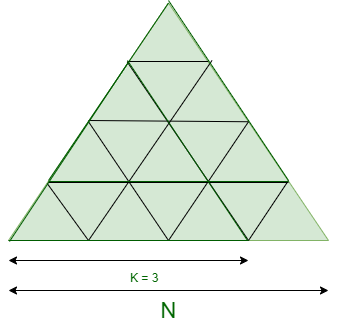Skip to content
Related Articles
Maximum count of Equilateral Triangles that can be formed within given Equilateral Triangle
• Difficulty Level : Expert
• Last Updated : 23 Mar, 2021

Given two integers N and K where N denotes the unit size of a bigger Equilateral Triangle, the task is to find the number of an equilateral triangle of size K that are present in the bigger triangle of side N.

Examples:

Input: N = 4, K = 3Output: 3
Explanation:
There are 3 equilateral triangles of 3 unit size which are present in the Bigger equilateral triangle of size 4 units.

Input: N = 4, K = 2
Output: 7
Explanation:
There are 7 equilateral triangles of 2 unit size which are present in the Bigger equilateral triangle of size 4 units.

Naive Approach: The idea is to iterate over all possible sizes of the bigger equilateral triangle for checking the number of triangles with the required size K and print the total count of triangles.

Time Complexity: O(N)
Auxiliary Space: O(1)

Efficient Approach: To optimize the above approach, observe the following points:

• The number of triangles with a peak in the upward direction of size K present in size N equals to ((N – K +1 ) * (N – K + 2))/2.
• The number of inverted triangles with a peak in the downward direction of size K present in size N equals to ((N – 2K + 1) * (N – 2K + 2))/2.

Below is the implementation of the above approach:

## C++

 `// C++ program for the above approach``#include ``using` `namespace` `std;` `// Function to find the number of``// equilateral triangle formed``// within another triangle``int` `No_of_Triangle(``int` `N, ``int` `K)``{``    ``// Check for the valid condition``    ``if` `(N < K)``        ``return` `-1;` `    ``else` `{` `        ``int` `Tri_up = 0;` `        ``// Number of triangles having``        ``// upward peak``        ``Tri_up = ((N - K + 1)``                  ``* (N - K + 2))``                 ``/ 2;` `        ``int` `Tri_down = 0;` `        ``// Number of inverted triangles``        ``Tri_down = ((N - 2 * K + 1)``                    ``* (N - 2 * K + 2))``                   ``/ 2;` `        ``// Total no. of K sized triangle``        ``return` `Tri_up + Tri_down;``    ``}``}` `// Driver Code``int` `main()``{``    ``// Given N and K``    ``int` `N = 4, K = 2;` `    ``// Function Call``    ``cout << No_of_Triangle(N, K);``    ``return` `0;``}`

## Java

 `// Java program for the above approach``import` `java.util.*;``class` `GFG{` `// Function to find the number of``// equilateral triangle formed``// within another triangle``static` `int` `No_of_Triangle(``int` `N, ``int` `K)``{``    ``// Check for the valid condition``    ``if` `(N < K)``        ``return` `-``1``;` `    ``else``    ``{``        ``int` `Tri_up = ``0``;` `        ``// Number of triangles having``        ``// upward peak``        ``Tri_up = ((N - K + ``1``) * (N - K + ``2``)) / ``2``;` `        ``int` `Tri_down = ``0``;` `        ``// Number of inverted triangles``        ``Tri_down = ((N - ``2` `* K + ``1``) *``                    ``(N - ``2` `* K + ``2``)) / ``2``;` `        ``// Total no. of K sized triangle``        ``return` `Tri_up + Tri_down;``    ``}``}` `// Driver Code``public` `static` `void` `main(String[] args)``{``    ``// Given N and K``    ``int` `N = ``4``, K = ``2``;` `    ``// Function Call``    ``System.out.print(No_of_Triangle(N, K));``}``}` `// This code is contributed by PrinciRaj1992`

## Python3

 `# Python3 program for the above approach` `# Function to find the number of``# equilateral triangle formed``# within another triangle``def` `No_of_Triangle(N, K):``  ` `    ``# Check for the valid condition``    ``if` `(N < K):``        ``return` `-``1``;` `    ``else``:``        ``Tri_up ``=` `0``;` `        ``# Number of triangles having``        ``# upward peak``        ``Tri_up ``=` `((N ``-` `K ``+` `1``) ``*``                  ``(N ``-` `K ``+` `2``)) ``/``/` `2``;` `        ``Tri_down ``=` `0``;` `        ``# Number of inverted triangles``        ``Tri_down ``=` `((N ``-` `2` `*` `K ``+` `1``) ``*``                    ``(N ``-` `2` `*` `K ``+` `2``)) ``/``/` `2``;` `        ``# Total no. of K sized triangle``        ``return` `Tri_up ``+` `Tri_down;``    ` `# Driver Code``if` `__name__ ``=``=` `'__main__'``:``    ``# Given N and K``    ``N ``=` `4``; K ``=` `2``;` `    ``# Function Call``    ``print``(No_of_Triangle(N, K));` `# This code is contributed by sapnasingh4991`

## C#

 `// C# program for the above approach``using` `System;``class` `GFG{` `// Function to find the number of``// equilateral triangle formed``// within another triangle``static` `int` `No_of_Triangle(``int` `N, ``int` `K)``{``    ``// Check for the valid condition``    ``if` `(N < K)``        ``return` `-1;` `    ``else``    ``{``        ``int` `Tri_up = 0;` `        ``// Number of triangles having``        ``// upward peak``        ``Tri_up = ((N - K + 1) * (N - K + 2)) / 2;` `        ``int` `Tri_down = 0;` `        ``// Number of inverted triangles``        ``Tri_down = ((N - 2 * K + 1) *``                    ``(N - 2 * K + 2)) / 2;` `        ``// Total no. of K sized triangle``        ``return` `Tri_up + Tri_down;``    ``}``}` `// Driver Code``public` `static` `void` `Main(String[] args)``{``    ``// Given N and K``    ``int` `N = 4, K = 2;` `    ``// Function Call``    ``Console.Write(No_of_Triangle(N, K));``}``}` `// This code is contributed by Rajput-Ji`

## Javascript

 ``
Output:
`7`

Time Complexity: O(1)
Auxiliary Space: O(1)

Attention reader! Don’t stop learning now. Get hold of all the important DSA concepts with the DSA Self Paced Course at a student-friendly price and become industry ready.  To complete your preparation from learning a language to DS Algo and many more,  please refer Complete Interview Preparation Course.

In case you wish to attend live classes with industry experts, please refer DSA Live Classes

My Personal Notes arrow_drop_up